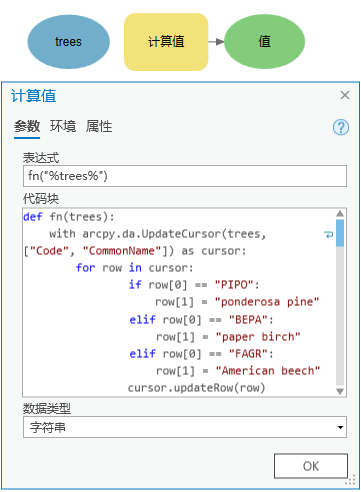# 使用计算值的示例

## 表达式

### 数学计算

x + y

x 加上 y

3 + 5

8

x - y

x 减去 y

4.3 - 2.1

2.2

x * y

x 乘以 y

8 * 9

72

x / y

x 除以 y

4 / 1.25

3.2

x // y

x 除以 y（向下取整除法）

4 // 1.25

3

x % y

x 除以 y 的余数

4 % 1.25

0.25

x**y

2 ** 3

8

x < y

2 < 3

1

x <= y

2 <=3

1

x > y

2 > 3

0

x >= y

2 >= 3

0

x == y

2 == 3

0

x != y

2 != 3

1

``````Expression:
math.sqrt(25)``````

``````Expression:
math.cos(0.5)``````

math 模块还支持常量。

``````Expression:
math.pi``````

``````Expression:
random.randint(0, 10)``````

``````Expression:
random.normalvariate(10, 3)``````

### 字符串示例

Python 运算符和索引可用于字符串值。

"Input" + " " + "Name"

"Input_Name"[6:]

"STREET".lower()

"Street Name".split()

``````Expression:
"%Value%".replace(".", "")``````

• 使用正斜线 (/) 代替反斜线杠。
• 使用两个反斜线代替一个。
• 通过在字符串前放置字母 r 将字符串转换为字符串文字。

## 数据类型

``````Expression:
%A% + 120``````

## 代码块

``````Expression:
5 + size

Code Block:
import os
size = 0
folderpath = r"C:\temp\csvFiles"
for ele in os.scandir(folderpath):
size += 1``````

### 时间

``````Expression:
gettime()

Code Block:
import time

def gettime():
# First replace removes punctuation marks. Second replace removes spaces.
return time.ctime().replace(":", "").replace(" ", "")``````

### If-then-else 和行内替换

``````Expression:
getAspectDir("%Input Degree%")

Code Block:
def getAspectDir(inValue):
inValue = int(inValue)
if inValue >= 45 and inValue < 135:
return "East"
elif inValue >= 135 and inValue < 225:
return "South"
elif inValue >= 225 and inValue < 315:
return "West"
else:
return "North"``````

### 多个行内替换

``````Expression:
fn("%User Input Value%","%Default Value%")

Code Block:
def fn(userInputValue, defaultValue):
if float(userInputValue) > float(defaultValue):
return float(userInputValue)
else:
return float(defaultValue)``````

### 数据路径串连

``````Expression:
getPath(r"%Folder Path%", "%Folder Name%")

Code Block:
import os

def getPath(folderPath, folderName):
outPath = os.path.join(folderPath, folderName)
if not os.path.exists(outPath):
os.makedirs(outPath)
return outPath``````

### 缓冲距离值和单位串联

• 将在代码块参数中返回一个距离值和一个线性单位。
• 数据类型参数值设置为线性单位

``````Expression:
fn("%A%", "%B%")

Code Block:
def fn(a, b):
distance = int(a) * int(b)
return f"{distance} Kilometers"``````

### ArcPy

ArcPyEsri Python 站点包，可提供通过 Python 执行地理数据分析、转换和管理及地图自动化的实用且高效的方式。通过 ArcPy 可访问地理处理工具以及其他函数、类和模块，从而创建简单或复杂的工作流。

#### 游标

``````Expression:
fn("%trees%")

Code Block:
def fn(trees):
with arcpy.da.UpdateCursor(trees, ["Code", "CommonName"]) as cursor:
for row in cursor:
if row == "PIPO":
row = "ponderosa pine"
elif row == "BEPA":
row = "paper birch"
elif row == "FAGR":
row = "American beech"
cursor.updateRow(row)``````#### 地理处理工具

ArcPy 还提供了对地理处理工具的访问。 可以在代码块参数中调用地理处理工具。

``````Expression:
fn("%Input Feature Class%")

Code Block:
def fn(InputFC):
# To allow overwriting outputs change overwriteOutput option to True.
arcpy.env.overwriteOutput = True

# Process: Select Layer By Attribute (Select Layer By Attribute) (management)
InputFC_Layer, Count = arcpy.management.SelectLayerByAttribute(InputFC, "NEW_SELECTION", "LABEL LIKE '%HIGHWAY%'")

# Process: Copy Features (Copy Features) (management)
copyFeaturesOutput = "C:\\temp\\Output.gdb\\copyFeaturesOutput"
arcpy.management.CopyFeatures(InputFC_Layer, copyFeaturesOutput)

# Process: Buffer (Buffer) (analysis)
bufferOutput = "C:\\temp\\Output.gdb\\bufferOutput"
arcpy.analysis.Buffer(copyFeaturesOutput, bufferOutput, "1500 Feet")
return bufferOutput``````